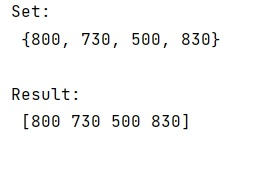# How to Convert a Set to a NumPy Array?

Given a Python set, we have to convert it into a NumPy array.
Submitted by Pranit Sharma, on March 02, 2023

NumPy is an abbreviated form of Numerical Python. It is used for different types of scientific operations in python. Numpy is a vast library in python which is used for almost every kind of scientific or mathematical operation. It is itself an array which is a collection of various methods and functions for processing the arrays.

## Converting a Set to a NumPy Array

Suppose that we are given some values on which we perform some operation and store the result into a set that we need to convert into a numpy array.

The set is one of the 4 built-in data types in Python and is used to store heterogeneous types of elements. A set can not contain repeated values hence the occurrence of any value in a set is always 1.

For this purpose, we will first convert the set into a list using the list function and then we will convert this list into a numpy array using the array function.

Let us understand with the help of an example,

## Python Code to Convert a Set to a NumPy Array

```# Import numpy
import numpy as np

# Defining some values
vals = np.array([50,80,73,83])

# Performing some operation and
# storing result in a set
s = set(vals*10)

# Display set
print("Set:\n",s,"\n")

# Converting set into numpy array
arr = np.array(list(s))

# Display result
print("Result:\n",arr,"\n")
```

Output:Languages: » C » C++ » C++ STL » Java » Data Structure » C#.Net » Android » Kotlin » SQL
Web Technologies: » PHP » Python » JavaScript » CSS » Ajax » Node.js » Web programming/HTML
Solved programs: » C » C++ » DS » Java » C#
Aptitude que. & ans.: » C » C++ » Java » DBMS
Interview que. & ans.: » C » Embedded C » Java » SEO » HR
CS Subjects: » CS Basics » O.S. » Networks » DBMS » Embedded Systems » Cloud Computing
» Machine learning » CS Organizations » Linux » DOS
More: » Articles » Puzzles » News/Updates